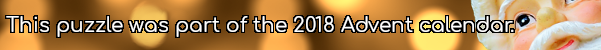mscroggs.co.uk
mscroggs.co.uksubscribeThe equation $$x^2+1512x+414720=0$$ has two integer solutions.
Today's number is the number of (positive or negative) integers $$b$$ such that $$x^2+bx+414720=0$$ has two integer solutions.